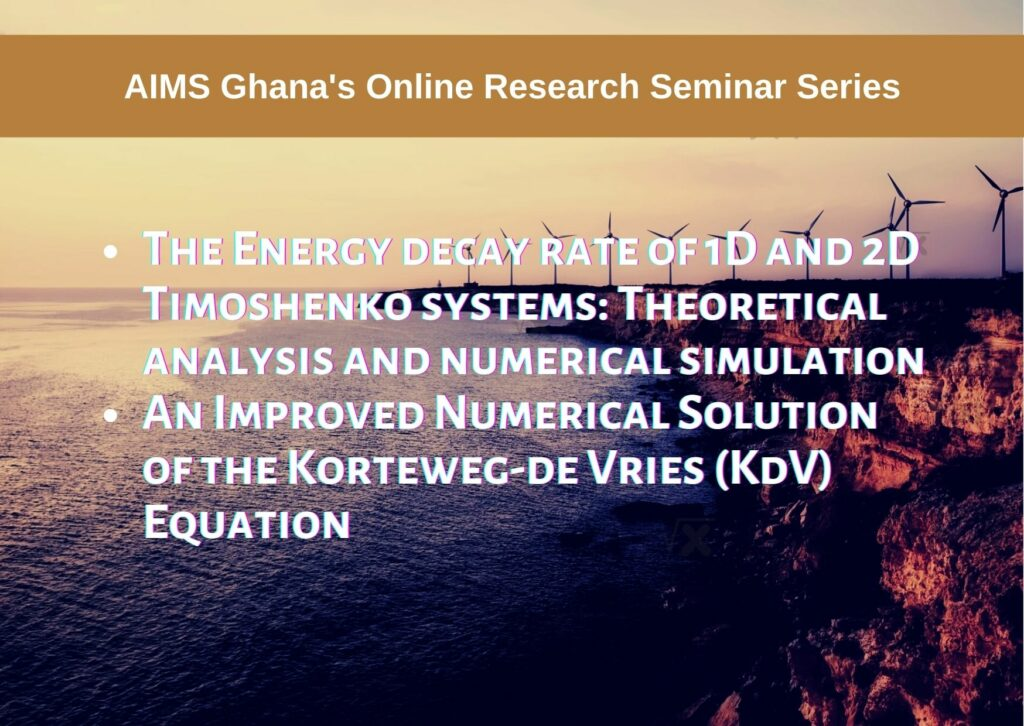November 18, 2021

# The Energy decay rate of 1D and 2D Timoshenko systems: Theoretical analysis and numerical simulation / An Improved Numerical Solution of the Korteweg-de Vries (KdV) Equation

Speaker 1: Dr. Sabrine Chebbi (Department of mathematics, University of Tunis El MANAR, UTM)

Title: The Energy decay rate of 1D and 2D Timoshenko systems: Theoretical analysis and numerical simulation

Abstract 1: We focus on the behavior of the solutions of the Timoshenko systems in dimensions 1 and 2 using the lower bound estimates of the energy. We study a nonlinear damped one-dimensional thermo-elastic Timoshenko system where the heat flux is given by Cattaneo’s law. After deriving the strong lower energy estimates, we show the optimality for some explicit examples. Furthermore, we discuss the well-posedness and the decay rate of the energy of the Mindlin-Timoshenko plate equations subject to a nonlinear dissipation acting on the rotation angles’ equations. Later, we perform a numerical simulation of the one-dimensional Timoshenko system, our approach is based on an adequate use of the finite element and the finite difference methods giving rise to a new scheme which characterizes the asymptotic behavior of the discrete energy.

Speaker 2: Kitavi Makaa Dominic (Department of Mathematics and Statistics, University of Embu, Kenya)

Short biography of Speaker: D. M. Kitavi obtained the B.Sc. (first-class honors) in Mathematics in 2011 from the University of Nairobi (Kenya), M.Sc. (cum laude) in Mathematical Sciences in 2013 from the University of the Western Cape (South Africa), and Ph.D. in Applied Mathematics in 2017 from the Hong Kong Polytechnic University (Hong Kong). D. M. Kitavi joined University of Embu as a lecturer in September 2017. D. M. Kitavi was appointed the Chairman/Head of Department of Mathematics and Statistics at the University of Embu in July 2021. D. M. Kitavi’s research interest lies in Partial Differential Equations and Statistical Signal Processing.

Title: An Improved Numerical Solution of the Korteweg-de Vries (KdV) Equation

Abstract 2: It has been a major challenge to identify methods which accurately solve certain non-linear Partial Differential Equations (PDEs). It should be understood that obtaining exact solutions of nonlinear PDEs, especially those with variable coefficients, may not be possible. This makes it necessary for mathematicians to devise ways/methods which can be used to, at least, get approximate solutions to such equations. Such methods, used to get approximate solutions to the non-linear PDEs, are referred to as numerical methods. The most commonly used numerical methods are Finite difference methods, Finite volume methods, Finite element methods, Method of lines, Spectral method, Domain decomposition methods, Mesh free methods, and Multigrid methods. Each of these methods has its own advantage and not all these listed methods can be applied to the same equation. Some methods are preferred at different situations while others cannot be applied in some situations. In the case of the KdV equation, I hereby consider finite difference methods which are easier to understand and bring out clearly the mathematical properties associated with the solution of the equation. The project numerically obtains an approximate solution to the KdV equation, whose exact solution is unavailable in the open literature.

Coordinator: Prof. Marc Sedjro (German Research Chair, AIMS South Africa)
Meeting ID: 926 0538 0740
Passcode: 107706 Zoom Meeting Start Time: 2:45pm (Accra Time)

NB: This meeting will not be recorded!
We kindly urge all participants to mute themselves and turn off their cameras when they join the meeting.

NB: Each talk is  30 minutes long + 15 minutes Questions and Answers session. Participants can ask their questions by any or a combination of the following:

1. By typing their questions in the chat box.

2. By writing “I have a question(s)” in the chat box.

3. Raising hands by clicking on the raise hand symbol.

The coordinator would then read out the questions to the presenter or ask the participant to ask his/her question(s).